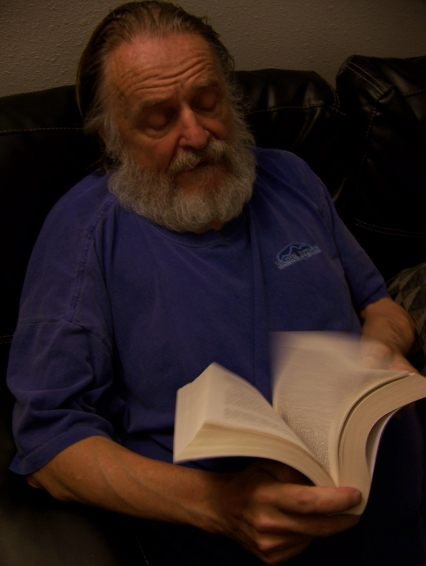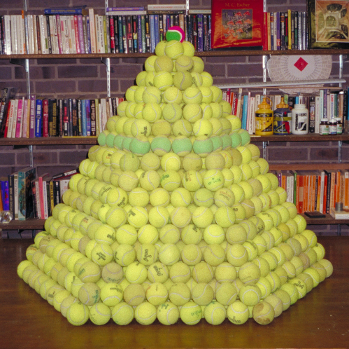## Genius at PlayThe last time I visited John Horton Conway, he showed me a book, Genius At Play: The Curious Mind of John Horton Conwayby Siobhan Roberts. It had nothing to do with him wanting to brag about the book. Nothing, nothing at all.He just wanted to show me a picture from the book. While he was browsing this book about himself, I took a photo of him (featured on the left). The picture he was looking for was on page 314. I am reproducing it here (Photo by Michael A. Stecker, courtesy of Stephen D. Miller).

When we finally found the picture, he asked me how many tennis balls were in it. I smelled a trick question right away and didn’t bite. I shrugged. It appears that one of the balls at the far corner at the base of the pyramid had rolled away. So there was one less ball than I would have calculated. So, how many balls are there?

Share:

1662?

2. #### Naveen Dankal:

down vote accepted

There are two different kinds of layers here, as it alternates between centered hexagonal numbers (e.g. the top layer is the first centered hexagonal number, i.e. 1, the third layer from the top is the second centered hexagonal number, i.e. 7, etc.), and layers where the sides alternate between lengths n and n+1 (e.g. the second layer from the top of the pyramid is a ‘hexagon’ with sides 1 and 2, the fourth layer is a hexagon with sides 2 and 3, etc.

So, the numbers of tennis balls in all the odd layers of this pyramid is 9^3=729

For the other layers: The formula for the figure with sides n and n+1 is 3n^2.

Since you have 8 of those layers, you have 3 times the sum of the first 8 square numbers, and the general sum of the first n square numbers is n(n+1)(2n+1)/6, so that gives you 3⋅8(8+1)(2⋅8+1)/6=612 more tennis balls, for a total of 729+612=1341